# Frequency assignment¶

The area of telecommunications, and in particular mobile telecommunications, gives rise to many different variants of frequency assignment problems. We are given a network of cells (nodes) with requirements of discrete frequency bands. Each cell has a given demand for a number of frequencies (bands). Figure 11.2.1 shows the structure of the network. Nodes linked by an edge are considered as neighbors. They must not be assigned the same frequencies to avoid interference. Furthermore, if a cell uses several frequencies they must all be different by at least 2. The objective is to minimize the total number of frequencies used in the network.

The following table lists the number of frequency demands for every cell:

 Cell 1 2 3 4 5 6 7 8 9 10 Demand 4 5 2 3 2 4 3 4 3 2

## Model formulation¶

Let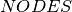be the set of all nodes in the network and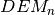the demand of frequencies at node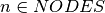. The network is given as a set of edges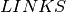. Furthermore, let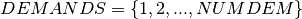be the set of frequencies, numbered consecutively across all nodes where the upper bound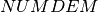is given by the total number of demands. The auxiliary array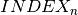indicates the starting index in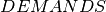for node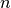. For representing the frequency assigned to every demand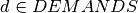we introduce the variables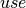that take their values from the set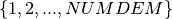. The two sets of constraints (different frequencies assigned to neighboring nodes and minimum distance between frequencies within a node) can then be modeled as follows.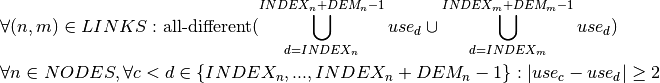The objective function is to minimize to the number of frequencies used. We formulate this by minimizing the largest frequency number that occurs for thevariables: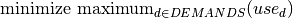## Implementation¶

The edges forming the telecommunications network are modeled as a list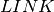, where edgeis given as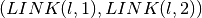. For the implementation of the constraints on the values of frequencies assigned to the same node we have two equivalent choices with Kalis, namely using abs or distance constraints.

const int NODES = 10;
// Range of links between nodes
const int LINKS = 18 ;

// Demand of nodes
int DEM[] = {4, 5, 2, 3, 2, 4, 3, 4, 3, 2};
// Neighboring nodes
int LINK = {{1, 3}, {1, 4}, {1, 6},{2, 4}, {2, 7},{3, 4}, {3, 6}, {3, 8}, {3, 9},{4, 7}, {4, 9}, {4,10},{5, 7}, {5, 8}, {5, 9},{6, 9}, {7, 8}, {8,10}};
// Start index in 'use'
int INDEX;
// Upper bound on no. of freq.
int NUMDEM;

int n;
NUMDEM = 0;
for (n=0;n<NODES;n++) {
NUMDEM += DEM[n];
}

// Correspondence of nodes and demand indices:
// use[d] d = 0, ..., DEM correspond to the demands of node 0
// d = DEM+1, ..., DEM+DEM(1)) - " - node 2 etc.

INDEX = 0;
for (n=1;n<NODES;n++) {
INDEX[n] = INDEX[n-1] + DEM[n-1];
}

// Creation of the problem in this session
KProblem problem(session,"Frequency assignment");

char name;
// Frequency used for a demand
KIntVarArray use;
int d,c;
for (d=0;d<NUMDEM;d++) {
sprintf(name,"use(%i)",d);
use += (* new KIntVar(problem,name,1,NUMDEM) );
}

// All frequencies attached to a node must be different by at least 2
for (n=0;n<NODES;n++) {
for (c=INDEX[n];c<= INDEX[n] + DEM[n] - 1;c++) {
for (d=INDEX[n];d<= INDEX[n] + DEM[n] - 1;d++) {
if (c < d) {
problem.post(KDistanceGreaterThanXyc(use[c],use[d],2));
}
}
}
}
// Neighboring nodes take all-different frequencies
int l;
KIntVarArray diff;
diff += use[d];
}
diff += use[d];
}
problem.post(KAllDifferent("diff",diff,KAllDifferent::USING_GCC));
}

// Objective function: minimize the number of frequencies used, that is
//minimize the largest value assigned to 'use'
problem.post(KMax("maxuse",numfreq,use,false));

// propagating problem
if (problem.propagate()) {
printf("Problem is infeasible\n");
exit(1);
}

// Search strategy
KBranchingSchemeArray myBa;
myBa += KAssignVar(KSmallestDomain(),KMinToMax(),use);

// Solve the problem
// creation of the solver
KSolver solver(problem,myBa);
solver.setDblControl(KSolver::MaxComputationTime,1.0);
solver.setSolutionFunctionPtr(solution_found,&problem);
// Try to find solution(s) with strategy 1
problem.setSense(KProblem::Minimize);
problem.setObjective(numfreq);
int nsol = -1;
if (solver.optimize())  {
solver.printStats();
KSolution * sol = &problem.getSolution();
nsol = sol->getObjectiveValue();
numfreq.setSup(nsol-1);
}
printf("Number of frequencies: %f\n", problem.getSolution().getObjectiveValue());
printf("Frequency assignment: \n");
for (n=0;n<NODES;n++) {
printf("Node %i: ",n);
for (d=INDEX[n];d<=INDEX[n]+DEM[n]-1;d++) {
printf("%i ",problem.getSolution().getValue(use[d]));
}
printf("\n");
}

// Search strategy
KBranchingSchemeArray myBa2;
myBa2 += KAssignVar(KMaxDegree(),KMinToMax(),use);

// Solve the problem
// creation of the solver
KSolver solver2(problem,myBa2);
solver2.setSolutionFunctionPtr(solution_found,&problem);
// Try to find solution(s) with strategy 2

if (solver2.optimize())  {
solver2.printStats();
KSolution * sol = &problem.getSolution();
}
printf("Number of frequencies: %f\n", problem.getSolution().getObjectiveValue());
printf("Frequency assignment: \n");
for (n=0;n<NODES;n++) {
printf("Node %i: ",n);
for (d=INDEX[n];d<=INDEX[n]+DEM[n]-1;d++) {
printf("%i ",problem.getSolution().getValue(use[d]));
}
printf("\n");
}


With just the default search strategy this model finds a solution of value 11 but it runs for a long time without being able to prove optimality. When experimenting with different search strategies we have found that the strategy obtained by changing the variable selection criterion to KMaxDegree is able to prove optimality easily once a good solution is known. This problem is therefore solved in two steps: First, we use the default strategy for finding a good solution. This search is stopped after one second by setting a time limit. The search is then restarted with a second strategy and the bound on the objective value from the previous run. Another new feature demonstrated by this implementation is the use of a callback, more precisely the solution callback of Artelys Kalis. The solution callback is defined with a user subroutine that will be called by the solver whenever the search has found a solution. Its typical uses are logging or storing of intermediate solutions or performing some statistics. Our procedure solution_found() simply prints out the solution that has been found.

int solution_found(void *param) {
KProblem * p = (KProblem *) param;
p->getSolution().printResume();
return 0;
}


## Improving the problem formulation¶

We may observe that in our problem formulation all demand variables within a node and the constraints on these variables are entirely symmetric. In the absence of other constraints, we may reduce these symmetries by imposing an order on thevariables,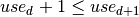for demands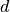and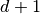belonging to the same cell. Doing so, the problem is solved to optimality within less than 40 nodes using just the default strategy. We may take this a step further by writing: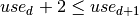. The addition of these constraints shortens the search by yet a few more nodes. They can even be used simply in replacement of the abs or distance constraints.

## Results¶

An optimal solution to this problem uses 11 different frequencies. The model shown in the program listing prints out the following assignment of frequencies to nodes: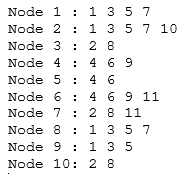Fig. 26 Assignment of frequencies result